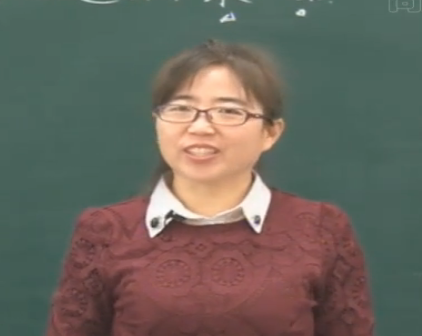尊敬的用户：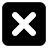三年级数学上册第四章《乘与除》练习

• 同步课程
• 课后作业
• 同步试卷
• 本节重点
• 主讲老师

《乘与除》练习

5.填一填。
（1）
×  30 40 80
2   60 80 160
6 180 240 480
7 210 280 560
（2）
×   20 40 50
3   60 120 150
5  100 200 250
8  160 320 400
6.小兔记录了自己4天采蘑菇的数量如下。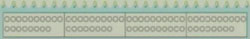7.苹果每箱17元 梨每箱15元
（1）买4箱苹果和6向梨，分别需要多少元？
（2）幼儿园买了3箱苹果和3箱梨，一共需要多少元？
（1）17×4=68（元）15×6=90（元）

（2）17×3+15×3=96（元）

（17+15）×3=96（元）

8.小东的爸爸和妈妈今年分别是多少岁？

14×3=42（岁）
14×2+10=38（岁）

9.买3个足球。

（100-7）÷3
=93÷3
=31（元）
10.松树每捆4棵40元

（1）每棵柏树多少元？
（2）一棵松树与一棵柳树比，哪个贵，贵多少元？
（3）三（1）班用全班同学捐的100元买了6棵柏树，还剩多少元？剩下的钱正好买了4棵杨树，

（1）36÷3=12（元）
（2）40÷4=10（元）
10＞8
10-8=2（元）
（3）12×6=72（元）
100-72=28（元）
28÷4=7（天）

一、填空题

1．在横线上填入“＞”“＜”或“=”。
16×44×16    200÷4200÷5  28÷128×1    3×124×9
560÷780×2   360÷3240÷2  450÷9540÷9  120÷360×2

【参考答案】：=、＞、=、=、＜、=、＜、＜

二、主观题

1．算一算，照样子分别再写出一组算式。
5×8=
5×80=
5×800=

28÷4=
280÷4=
2800÷4=

【参考答案】：
 5×8=40 5×80=400 5×800=4000 3×4=12 3×40=120 3×400=1200 28÷4=7 280÷4=70 2800÷4=700 24÷6=4 240÷6=40 2400÷6=400
（右边两框答案不唯一）

2．买冷饮。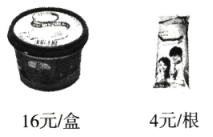(1)买3盒冰淇淋和5根雪糕分别需要多少元？
(2)李阿姨想买4盒冰淇淋和4根雪糕，一共需要多少元？（请用两种不同的方法解答）
(3)买6根雪糕的钱，刚好可以买8瓶汽水，1瓶汽水多少元？

【参考答案】：(1)16×3=48（元）  4×5=20（元）

(2)方法一：16×4+4×4=80（元）

(3)4×6=24（元）  24÷8=3（元）

3．根据销售小票算一算，1双保暖拖鞋多少元？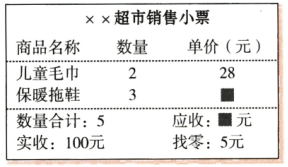【试题解析】：这是一道综合题。首先在销售小票中找到“实收：100元”“找零：5元”，可用减法算出实际花了95元。95元是2条儿童毛巾和3双保暖拖鞋的钱，从中减去儿童毛巾的钱，剩下的钱就是3双保暖拖鞋的钱，再除以保暖拖鞋的数量就是1双保暖拖鞋的价格。

【参考答案】：100-5=95（元）  95-28×2=39（元）
39÷3=13（元）